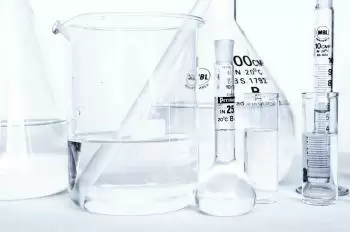# Intensive and Extensive Thermodynamic PropertiesA thermodynamic property is any property that defines a particular state of a physical system that can be measured.

The thermodynamic properties of a system can be classified as intensive and extensive properties. Those which do not depend on the amount of matter in the system (pressure, temperature, composition) are intensive. The extensive ones depend on the size of the system (mass, volume).

## What Is an Intensive Property in Thermodynamics?

Intensive properties are physical properties that do not depend on the quantity of matter or the system size. For example, if we divide a thermodynamic system into two parts, an intensive property maintains the same value in each piece.

For example, the density of water is the same if it is concentrated in a liter as it is concentrated in a vast reservoir.

This set includes all specific values ​​such as specific internal energy, specific enthalpy, specific entropy, temperature, pressure, specific volume, etc.

### Examples of Intensive Thermodynamic Properties

Here is a brief description of some of them:

• Density: density is the relationship between mass and the volume it occupies, that is, the mass per unit volume.

• Specific volume - We define specific volume as volume per unit mass.

• Specific weight - We define it as the relationship between the body's weight and the total volume it occupies.

• Heat capacity - It is the amount of heat that we need to increase the temperature of a given amount of matter by one kelvin.

• Pressure - Pressure is the force exerted by a body per unit area.

• Temperature - Temperature is the thermal state of the system that is considered to transmit heat.

## What Is an Extensive Property in Thermodynamics?

An extensive property is a property that depends on the amount of matter in a sample and the system's size. If a system is composed of different subsystems, the value of the entire extensive property will be the sum of the value of the various subsystems.

Extensive properties become intensive if they are expressed per unit of mass (specific property), of moles (molar property), or volume (property density).

This group of system properties includes total values, such as total energies, volume, weight, amount of substance, etc.

### Examples of Extensive Thermodynamic Properties

Here are some extensive thermodynamic properties:

• Heat: Heat is the energy that flows between two substances at different temperatures.

• Work: Work in thermodynamics always represents an exchange of energy between a system and its environment. By convention, the work done by the environment on the system has a positive sign.

• Internal energy: the internal energy of a system is the sum of all the existing energies in the system. This type of energy cannot be determined in absolute form. Therefore, what is measured is the variation of the system's internal energy.

Author:

Published: April 17, 2019
Last review: November 28, 2021